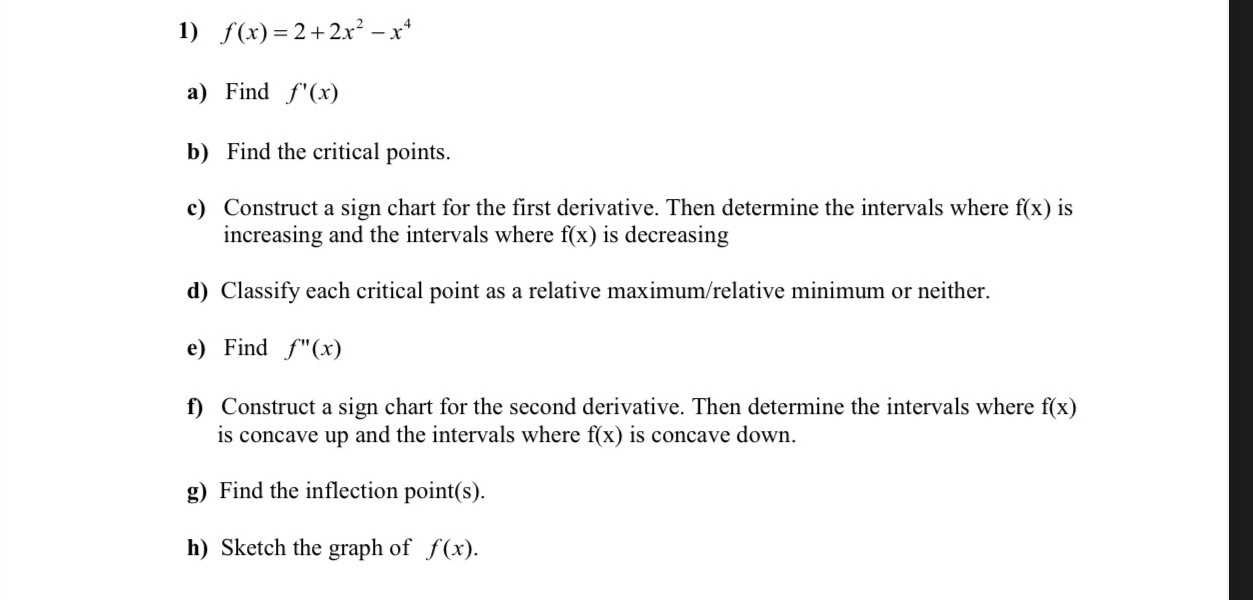### ¿Todavía tienes preguntas de matemáticas?

Pregunte a nuestros tutores expertos
Algebra
Pregunta1) $$f ( x ) = 2 + 2 x ^ { 2 } - x ^ { 4 }$$

a) Find $$f ^ { \prime } ( x )$$

b) Find the critical points.

c) Construct a sign chart for the first derivative. Then determine the intervals where $$f ( x )$$ is increasing and the intervals where $$f ( x )$$ is decreasing

d) Classify each critical point as a relative maximum/relative minimum or neither.

e) Find $$f ^ { \prime \prime } ( x )$$

f) Construct a sign chart for the second derivative. Then determine the intervals where $$f ( x )$$

is concave up and the intervals where $$f ( x )$$ is concave down.

g) Find the inflection point(s).

h) Find the inflection point(s).

a)

$$f ^ { \prime } ( x ) = 2 + 2 x ^ { 2 } - x ^ { 4 } : \quad f ( x ) = 2 x + \frac { 2 x ^ { 3 } } { 3 } - \frac { x ^ { 5 } } { 5 } + c _ { 1 }$$

b)

Critical Points of $$2 + 2 x ^ { 2 } - x ^ { 4 } : \quad x = - 1 , x = 0 , x = 1$$

e)

$$f ^ { \prime \prime } ( x ) = 2 + 2 x ^ { 2 } - x ^ { 4 } : \quad f ( x ) = x ^ { 2 } + \frac { x ^ { 4 } } { 6 } - \frac { x ^ { 6 } } { 30 } + c _ { 1 } x + c _ { 2 }$$

g)

Inflection Points of $$2 + 2 x ^ { 2 } - x ^ { 4 } : ( - \sqrt { \frac { 1 } { 3 } } , \frac { 23 } { 9 } ) : ( \sqrt { \frac { 1 } { 3 } } , \frac { 23 } { 9 } )$$

Solución
View full explanation on CameraMath App.